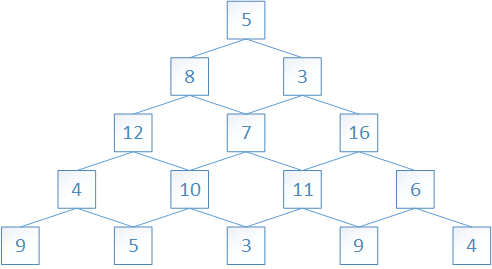# 动态规划

## 树塔问题##### 树塔问题示意图

dp[i][j] = max(dp[i+1][j],dp[i+1][j+1]) + f[i][j]

f[i][j]存放第i层的第j个数字,那么就有f=5,f=8 ...

dp[i][j]称为问题的状态，上面的式子称为状态转移方程 ,它把状态dp[i][j]转移为dp[i+1][j]dp[i+1][j+1]。可以发现，状态dp[i][j]只与第i+1层的状态有关，而与其他层的状态无关，这样层号为i的状态就总是可以由层号为i+1的两个子状态得到。那么，如果总是将层号增大，什么时候会到头呢？可以发现，树塔的最后一层的dp值总是等于元素本身，即dp[n][j] == f[n][j]，把这种可以直接确定其结构的部分称为边界 ，而动态规划的递推写法总是从这些边界出发，通过状态转移方程扩散到整个dp数组。

## 最大连续子序列

-2 11 -4 13 -5 -2

1. 令状态dp[i]表示以A[i]作为末尾的连续序列的最大和（这里说A[i]必须作为连续序的末尾）

2. 边界：$dp = A$

3. 状态转移方程： $dp[i]= max{A[i],dp[i-1]+A[i]}$